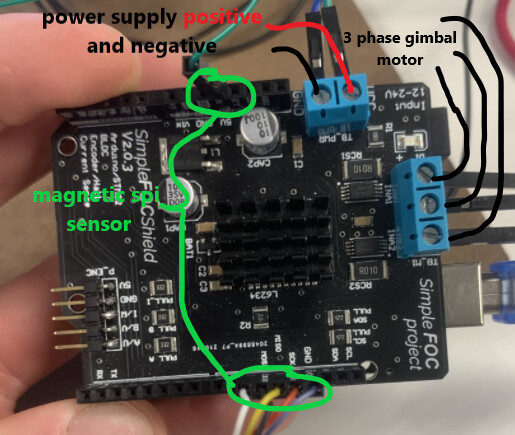# Unable to sense current

Hi all.
I have been trying to use current torque control for quite some time now, but I have never been able to get current sensing to work. I have used all the other example codes with great success (Thanks for all the great work by the way) but I am having trouble with anything involving current sensing. This leads me to believe the problem is something in the hardware. However, the hardware seems straight forward. I am somewhat new to the community so if someone with more experience than myself wouldn’t mind looking at my setup to see if there is anything wrong, I would greatly appreciate it.
My hardware is a GM4108 ipower gimble motor and a Simple FOC shield v2.0.3
Here is the setup.Here is the code.
#include <SimpleFOC.h>

// current sensor
// shunt resistor value
// gain value
// pins phase A,B
InlineCurrentSense current_sense = InlineCurrentSense(0.01, 50.0, A0, A2);
float current_magnitude;

LowPassFilter filter = LowPassFilter(0.001); // Tf = 1ms

void setup() {
// initialise the current sensing
current_sense.init();

// for SimpleFOCShield v2.01/v2.0.2
current_sense.gain_b *= -1;

Serial.begin(115200);
}

void loop() {
PhaseCurrent_s currents = current_sense.getPhaseCurrents();

``````current_magnitude = current_sense.getDCCurrent();
``````

filter.Tf = 0.01;
float filter_signal = filter(current_magnitude);

digitalWrite(5, LOW);
digitalWrite(6, LOW);
digitalWrite(9, LOW);
digitalWrite(8, HIGH);

// Serial.print(currents.a1000); // milli Amps
// Serial.print("\t");
// Serial.print(currents.b
1000); // milli Amps
//Serial.print("\t");
//Serial.print(currents.c1000); // milli Amps
// Serial.print("\t");
// Serial.println(current_magnitude
1000); // milli Amps
Serial.println(filter_signal); // milli Amps
}

This code only returns zero even when I back drive the motor.

There might be a bug in the lib causing random issues with current sensing. Please see this topic.